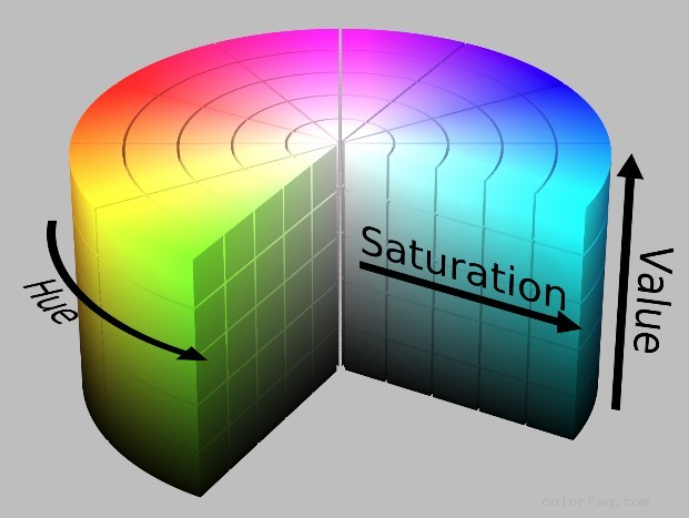HSL and RGB conversionHSL:

H: Hue tone

S: Saturation

L: Lightness

aRGB:

a: alpha transparency

R: Red

G: Green

B: Blue

a) RGB→HSL algorithm description.

Step 1: Convert the RGB value to the middle value of [0, 1].

Step 2: Find the maximum of R, G, and B.

Step 3: Calculate the brightness: L=(maxcolor + mincolor)/2

Step 4: If the maximum and minimum color values are the same, it means gray, then S is defined as 0, while H is undefined and is usually written as 0 in the program.

Step 5: Otherwise, calculate the saturation S according to the brightness L:

If L<0.5, S=(maxcolor-mincolor)/(maxcolor + mincolor)

If L>=0.5, S=(maxcolor-mincolor)/(2.0-maxcolor-mincolor)

Step 6: Calculate hue H:

If R=maxcolor, H=(G-B)/(maxcolor-mincolor)

If G=maxcolor, H=2.0+(B-R)/(maxcolor-mincolor)

If B=maxcolor, H=4.0+(R-G)/(maxcolor-mincolor)

H=H*60.0, if H is negative, add 360.

Explanation: 1. From the formula in step 3, it can be seen that the brightness is only related to the total amount of the most and least color components of the image. The lower the brightness, the more black the image becomes. The higher the brightness, the brighter the image tends to be white.

2. From the formula in step 5, it can be seen that the saturation is related to the difference between the most and least color components of the image. The smaller the saturation, the more the image tends to be a grayscale image. The greater the saturation, the brighter the image, which gives the impression of being colored rather than black, white and gray.

3. The color tone reflects the different color perceptions of the image.

4. From the calculation in step 6, H is divided into 0-6 areas. The RGB color space is a cube and the HSL color space is two hexagonal cones, where L is the main diagonal of the RGB cube. Therefore, the vertices of the RGB cube: red, yellow, green, cyan, blue, and magenta become the vertices of the HSL hexagon, and the value 0-6 tells us where the H is.

b) The algorithm description of HSL→RGB.

Step 1: If S=0, it means gray, and define R, G and B as L.

Step 2: Otherwise, test L:

If L<0.5,temp2=L*(1.0+S)

If L>=0.5,temp2=L+S-L*S

Step 3: temp1=2.0*L-temp2

Step 4: Convert H to 0～1.

Step 5: For R, G, B, calculate another temporary value temp3. Methods as below:

for R, temp3=H+1.0/3.0

for G, temp3=H

for B, temp3=H-1.0/3.0

if temp3<0, temp3=temp3+1.0

if temp3>1, temp3=temp3-1.0

Step 6: Do the following tests for R, G, and B:

If 6.0*temp3<1,color=temp1+(temp2-temp1)*6.0*temp3

Else if 2.0*temp3<1,color=temp2

Else if 3.0*temp3<2,

color=temp1+(temp2-temp1)*((2.0/3.0)-temp3)*6.0

Else color=temp1